# Electron Paramagnetic Resonance EPR

The following article is from The Great Soviet Encyclopedia (1979). It might be outdated or ideologically biased.

## Electron Paramagnetic Resonance (EPR)

the resonance absorption of electromagnetic energy in the centimeter or millimeter wavelength range by substances that contain paramagnetic particles. EPR is a method of radio-frequency spectroscopy. The following may be paramagnetic particles: atoms or molecules with—as a rule—an odd number of electrons, for example, nitrogen or hydrogen atoms or NO molecules; free radicals, such as CH3; ions with partially filled inner electron shells, for example, ions of transition elements; color centers in crystals; impurity atoms, such as donors in semiconductors; and conduction electrons in metals or semiconductors.

EPR was discovered by E. K. Zavoiskii in 1944. In 1922 the first works suggesting the possible existence of EPR appeared. An experimental detection of EPR was attempted in the mid-1930’s by the Dutch physicist C. Gorter and co-workers. However, EPR was observed only through the use of the radio-frequency spectroscopic methods that were developed by Zavoiskii.

EPR is a particular case of magnetic resonance. A description of EPR in the framework of classical physics consists in the following. In an applied static magnetic field H, the magnetic moment µ of a paramagnetic particle precesses about the magnetic field H at a frequency of v, which is given by the equation

(1) 2πv = γH

where γ is the gyromagnetic ratio. In this case, the angle of precession θ—that is, the angle between the vectors H and μ—remains constant. If the system is placed in a magnetic field H1H that rotates about H at a frequency of v, the projection of the vector µ on the direction of the field H changes at a frequency of v1 = γH1/2π. The change in the projection of µ at a frequency of V1 under the action of a radio-frequency field H1 (Figure 1) is resonant in nature and gives rise to EPR. In the investigation of EPR, a linearly polarized alternating magnetic field is usually employed. Such a field may be represented as the sum of two fields rotating in opposite directions at a frequency of v. One of the components, which rotates in the same direction as the precession, causes the change in the projection of the magnetic moment µ on H.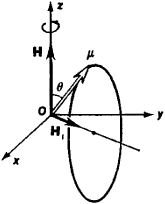Figure 1. Change in the angle θ of precession of a magnetic moment µ at a frequency of v1 = γH1/2π in a coordinate system Oxyz that rotates together with the field H1 about H at a frequency of v = γH/2π

The classical treatment is convenient for the analysis of relaxation processes (see below). However, a quantum approach is required to describe EPR spectra. Electromagnetic energy is absorbed in the case where a quantum of electromagnetic energy hv, where h is Planck’s constant, is equal to the energy difference Δℰ between the magnetic sublevels, or Zeeman sublevels, that are formed as a result of the splitting of the energy levels of a paramagnetic particle in a static magnetic field H (seeZEEMAN EFFECT).

If the magnetic moment of a paramagnetic particle is due only to electron spin s = ½, then µ = gsβMs, where gs = 2.0023 is the Landé splitting factor (the g-factor) for a free electron, β is the Bohr magneton, and Ms is the magnetic quantum number, which takes a value of ±½. In an applied static magnetic field H, the free electrons of paramagnetic particles are divided into two groups with energies of – gsβH/2 or + gsβH/2. Quantum transitions excited by a field H1H are possible between the two groups of energy levels. The resonance condition is written as

(2) hv = Δℰ = gsβH

Condition (2) is equivalent to resonance condition (1) since γ = 2πgsβ/h.

The distribution of electrons between two energy levels is described by Boltzmann’s equation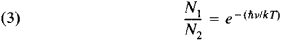where N1 is the number of electrons in the upper level, N2 is the number of electrons in the lower level, T is the temperature, and k is the Boltzmann constant. Under the action of an electromagnetic field H1, the electrons undergo transitions from one level to the other and the direction of the electron spins changes. Upon a transition from the lower level to the upper level, electromagnetic energy is absorbed; upon a transition from the upper level to the lower level, electromagnetic energy is emitted. The probabilities of the two processes are the same. However, under equilibrium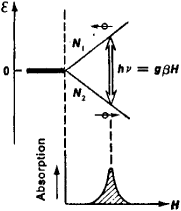Figure 2. Resonance absorption of the energy of an alternating electromagnetic field at hv = gβH

conditions, the population of the lower level is greater than that of the upper level. Therefore, energy is absorbed (Figure 2). If the population is inverted by some artificial means, the system will radiate energy under the action of an electromagnetic field. The principle of population inversion by artificial means is the basis of the operation of paramagnetic quantum mechanical amplifiers.

The paramagnetism of particles is usually due to the total contribution of the orbital and spin angular momenta of some electrons. In crystals, the electrons involved are also affected by the strong electric fields of surrounding ions or ligands. Therefore, in the case of crystals, the description of the structure of EPR spectra is a complicated problem. To compute such spectra, a semiempirical method called the spin Hamiltonian method is used. The method was proposed by A. Abragam (France) and M. H. L. Pryce (Great Britain) in 1951.

In EPR, transitions between closely spaced energy levels occur. The calculation of energy levels in a magnetic field is simplified if an effective spin S is introduced. The absolute value of S is determined by the number n of closely spaced levels: n = 2S + 1. The energies are calculated on the assumption that the magnetic moment of a particle is due to the quantity S. Then, the energy of a level is ℰ = gβMsH where Ms takes any of (2S + 1) values: S, (S – 1), . . ., –(S – 1), –S. The value of the g-factor may differ substantially from that of gs, the g-factor of a free electron. Dipole transitions are possible between levels that differ in Ms by ΔMS = ±1; as before, the resonance condition will be described by equation (2) but with gs = g. If S > ½, energy levels with different ǀMSǀ may be split when H = 0. In this case, several absorption lines appear in an EPR spectrum. The absorption lines constitute the fine structure of the spectrum (Figure 3,a).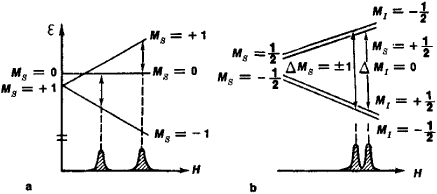Figure 3. (a) The fine structure of an EPR spectrum. For the case S = 1, two absorption lines are observed as a result of level splitting at H = 0. (b) The hyperfine structure of an EPR spectrum.

The interaction of electrons with the magnetic moment of the nucleus of a paramagnetic atom results in the appearance of hyperfine structure in an EPR spectrum. If the spin of the nucleus is I, the number of hyperfine components is equal to 2I + 1, corresponding to the condition for a transition, ΔMI = 0, where MI is the nuclear magnetic quantum number (Figure 3,b). The interaction of the electrons of a paramagnetic particle with the magnetic moments of the nuclei of surrounding ions also splits an EPR line, giving rise to superhyperfine structure (Figure 4). The study of hyperfine and superhyperfine interactions makes it possible to determine the distributions of unpaired electrons.

Paramagnetic relaxation.LINE WIDTHS. Relaxation processes restore equilibrium in a system of electron spins in which equilibrium is disturbed as a result of the absorption of electromagnetic energy. Such processes are characterized by two relaxation times, T1 and T2. The relation between the width Δv of absorption lines and the relaxation times is given by the equation

Δv = (1/T1) + (1/T2)

In the classical treatment, the times T1 and T2 are called the longitudinal relaxation time and the transverse relaxation time, respectively, because they specify the times required for the restoration of the equilibrium position of the longitudinal component and the transverse component, respectively, of the magnetization vector. The equilibrium value of the transverse component of the magnetization is restored owing to the interaction between the magnetic moments of paramagnetic particles, that is, owing to the spin-spin interaction. Hence, T2 is also called the spin-spin relaxation time. The restoration of the longitudinal component is due to the interaction of the magnetic moments of paramagnetic particles with lattice vibrations, that is, to the spin-lattice interaction. Therefore, the time T1 is also referred to as the spin-lattice relaxation time. It characterizes the rate of restoration of equilibrium between a spin system and lattice vibrations.

The spin-spin interaction consists of two components: a dipole interaction and an exchange interaction. The local field acting on a paramagnetic particle is composed of the applied field H and a field Hd that is generated by the dipoles, or magnetic moments, of neighboring paramagnetic particles. The field Hd varies from point to point because the assembly of neighboring paramagnetic particles and the directions of the magnetic moments of the particles vary, resulting in the broadening of an EPR line. On the other hand, the exchange interaction tends to orient the spin directions and, consequently, lessens the randomness of the orientations of the magnetic moments of paramagnetic particles. Therefore, the exchange interaction results in the exchange narrowing of an EPR line.

The motions of the nuclei of paramagnetic centers give rise to electric field fluctuations, which affect the orbital motion of electrons. In turn, the disturbance of the electrons’ orbital motion results in fluctuations of the local magnetic field and, consequently, in the broadening of EPR lines. The magnitude of the spin-lattice interaction decreases with temperature because the amplitude of the thermal vibrations of the lattice of nuclei is reduced. The magnitude of the spin-spin interaction is virtually independent of temperature. Therefore, for ions of transition metals and a large contribution of orbital angular momentum, an EPR line is observed only at low temperatures.

EPR spectra are observed at a rather low power of the alternating electromagnetic field (10–2–10–3 watt) in the case where the steady state differs little from the equilibrium state. If the power is high and the relaxation processes cannot restore the equilibrium distribution, the populations of the energy levels are equalized and saturation occurs; the saturation can be detected on the basis of decreased absorption (seeQUANTUM ELECTRONICS). The saturation effect is used to measure paramagnetic relaxation times.

Experimental methods. EPR is observed in the microwave region. The absorption of energy increases as the frequency increases because, in accordance with (3), the difference in the populations of energy levels increases as the frequency increases. A sufficiently high sensitivity is achieved at a frequency of v = 9,000 megahertz (MHz), which corresponds to H = 3,200 oersteds, that is, to a magnetic field strength that can be readily obtained under laboratory conditions. The use of powerful electromagnets and superconducting solenoids makes it possible to perform experiments at frequencies of up to v = 150,000 MHz (a wavelength of λ = 2 mm).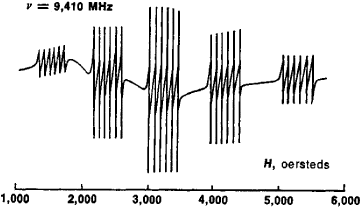Figure 4. The EPR spectrum of the Mn2+ ion in a metasilicate crystal. Five groups of lines constituting fine structure are seen; the groups correspond to the spin of the Mn2+ ion: S = 5/2. Each group consists of six lines constituting hyperfine structure, which is due to the interaction with the nuclear spin I = 5/2.

To measure absorption, radio-frequency spectrometers called EPR spectrometers are used. In EPR spectrometers, a change in the power absorbed in a specimen is recorded at a fixed frequency as the applied magnetic field is slowly varied.

In direct-amplification EPR spectrometers, microwaves generated by a klystron are supplied through a wave guide to a cavity resonator—that is, a cavity with a dimension of ~λ—placed between the poles of an electromagnet. Electromagnetic waves that pass through the resonator or that are reflected by it enter a crystal detector. A change in the power absorbed in the specimen is recorded on the basis of a change in the current of the detector. To increase the sensitivity of such a spectrometer, the applied magnetic field is modulated at a frequency of 30 hertz to 1 MHz. When the specimen absorbs power, microwaves that pass through the resonator or that are reflected by it also turn out to be modulated. The modulated signal is amplified, detected, and supplied to a recording device, such as an oscillograph or a strip-chart recorder. The recorded signal has the form of a derivative of the absorption curve (Figure 4). The sensitivity of the spectrometer is determined by the amplifier’s thermal noise level.

In superheterodyne spectrometers, power from an auxiliary klystron is supplied to the detector. The frequency of the oscillations generated by the auxiliary klystron differs from the frequency of the main klystron. The signal from the detector is amplified at a difference frequency of 30–100 MHz.

Applications. The best studied EPR spectra are those of ions of transition metals. To eliminate the line broadening due to the dipole interaction with neighboring paramagnetic ions, measurements are made using single crystals that are diamagnetic dielectrics into which paramagnetic ions are introduced as impurities (0.001–0.1 percent). The effect of the surrounding ions on a paramagnetic ion is treated as the effect of point charges. EPR is observed in the filled lower energy levels of a paramagnetic ion that result from the splitting of the ground state by the electric field of the surrounding charges (seeCRYSTAL FIELD).

In the case of ions of rare-earth elements, a crystal field turns out to be weak in comparison with the interaction of an ion’s electrons because the paramagnetism of such ions is due to deep-seated 4f electrons. The angular momentum of such an ion is determined by the sum of the orbital and spin angular momenta of the ground state. In a crystal field, levels with different absolute values of the projection of the bulk magnetic moment are not equivalent with respect to energy.

The paramagnetism of ions of the Fe group is due to 3d electrons. For such ions, a crystal field turns out to be stronger than the spin-orbit coupling, which determines the energy spectrum of a free ion. As a result, the maximum value of the projection of the orbital angular momentum either decreases or becomes equal to zero. This effect is usually referred to as the partial or complete “freezing” of the orbital angular momentum.

The symmetry of a crystal field determines the symmetry of the g-factor, and the crystal field strength determines the value of the g-factor. Therefore, the study of the g-factor of paramagnetic ions makes it possible to investigate crystal fields. The charge of a paramagnetic ion and the symmetry of the surrounding ions may also be determined from EPR spectra. Combined with data obtained by X-ray diffraction analysis, such determinations make it possible to specify the location of a paramagnetic ion in a crystal lattice. Knowledge of the energy levels of a paramagnetic ion makes it possible to compare EPR results with data on optical spectra and to calculate the magnetic susceptibilities of paramagnetic substances.

EPR is widely used in chemistry. During chemical reactions or upon exposure to ionizing radiation, molecules in which at least one electron is unpaired may be formed. Such molecules, called free radicals, are relatively stable and have a high chemical activity. Free radicals play a large role in the kinetics of chemical reactions, and EPR is one of the most important methods of investigating such radicals. The g-factor of free radicals is usually close to the value of g5; the line width for free radicals is small. Because of these characteristics, α-diphenyl-β-picrylhydrazyl, which is one of the most stable free radicals and for which g = 2.0036, is used as a standard in EPR measurements.

The study of localized unpaired electrons is of prime importance for investigations of mechanisms of tissue damage and of formation mechanisms for intermediates in enzymatic catalysis or other types of catalysis. Therefore, EPR is heavily used in biology to study enzymes, free radicals in biological systems, and metallo-organic compounds.

In crystals, delocalized electrons or holes may be trapped by defects or impurities, which are virtually unavoidable in a crystal lattice. Very often, such trap centers determine the color of a crystal (seeCOLOR CENTER). EPR makes it possible to determine the nature and position of color centers from the distribution of unpaired electrons. EPR due to electrons that are bound to donors can be observed in semiconductors.

In metals and semiconductors, two types of resonance phenomena involving conduction electrons are possible. The first is cyclotron resonance, which is due to changes in the orbital motion of conduction electrons under the influence of an alternating superhigh-frequency electric field. The second is EPR associated with changes in the orientations of the spins of conduction electrons. EPR involving conduction electrons is difficult to observe owing to the following: (1) the fraction of unpaired conduction electrons is small, ~kT/ℰF, where ℰF, is the Fermi energy; (2) because of the skin effect, the penetration depth of an electromagnetic field in the microwave band is extremely small, ~10–3–10–6cm; and (3) the absorption line shape is highly distorted because of the skin effect and electron diffusion.

EPR is observed in solutions and glasses that contain ions of transition metals, making it possible to determine, for example, the charge of paramagnetic ions and the structure of spheres of solvation. EPR spectra in gases—such as O2, NO, and NO2—are more complicated owing to spin-orbit coupling, the rotational motion of molecules, and the influence of nuclear spin.

### REFERENCES

Al’tshuler, S. A., and B. M. Kozyrev. Elektronnyi paramagnitnyi rezonans soedinenii elementov promezhutochnykh grupp, 2nd ed. Moscow, 1972.
Abragam, A., and B. Bleaney. Elektronnyi paramagnitnyi rezonans perekhodnykh ionov, vols. 1–2. Moscow, 1972–73. (Translated from English.)
Pake, G. E. Paramagnitnyi rezonans. Moscow, 1965. (Translated from English.)
Ballhausen, C. Vvedenie v teoriiu polia ligandov. Moscow, 1964. (Translated from English.)
Atkins, P., and M. Symons. Spektry EPR istroenie neorganicheskikh radikalov. Moscow, 1970. (Translated from English.)
Ingram, D. Elektronnyi paramagnitnyi rezonans v svobodnykh radikalakh. Moscow, 1961. (Translated from English.)
Ingram, D. Elektronnyi paramagnitnyi rezonans v biologii. Moscow, 1972. (Translated from English.)
Ludwig, G., and H. Woodbury. Elektronnyi spinovoi rezonans v poluprovodnikakh. Moscow, 1964. (Translated from English.)

V. F. MESHCHERIAKOV